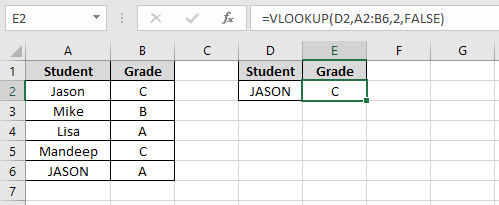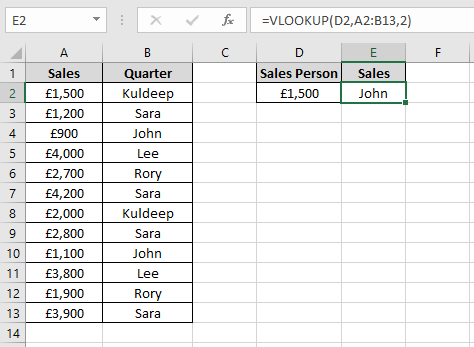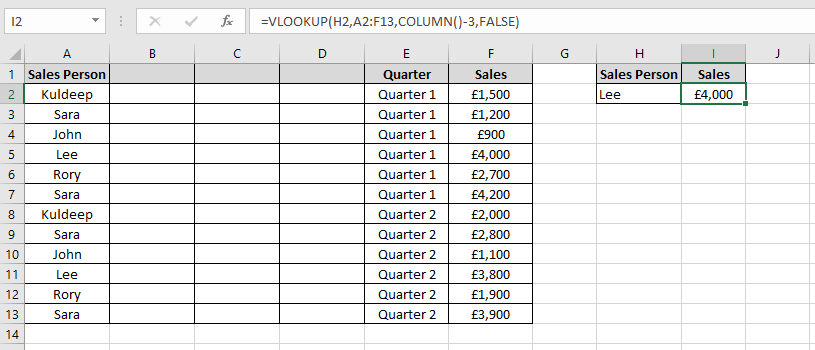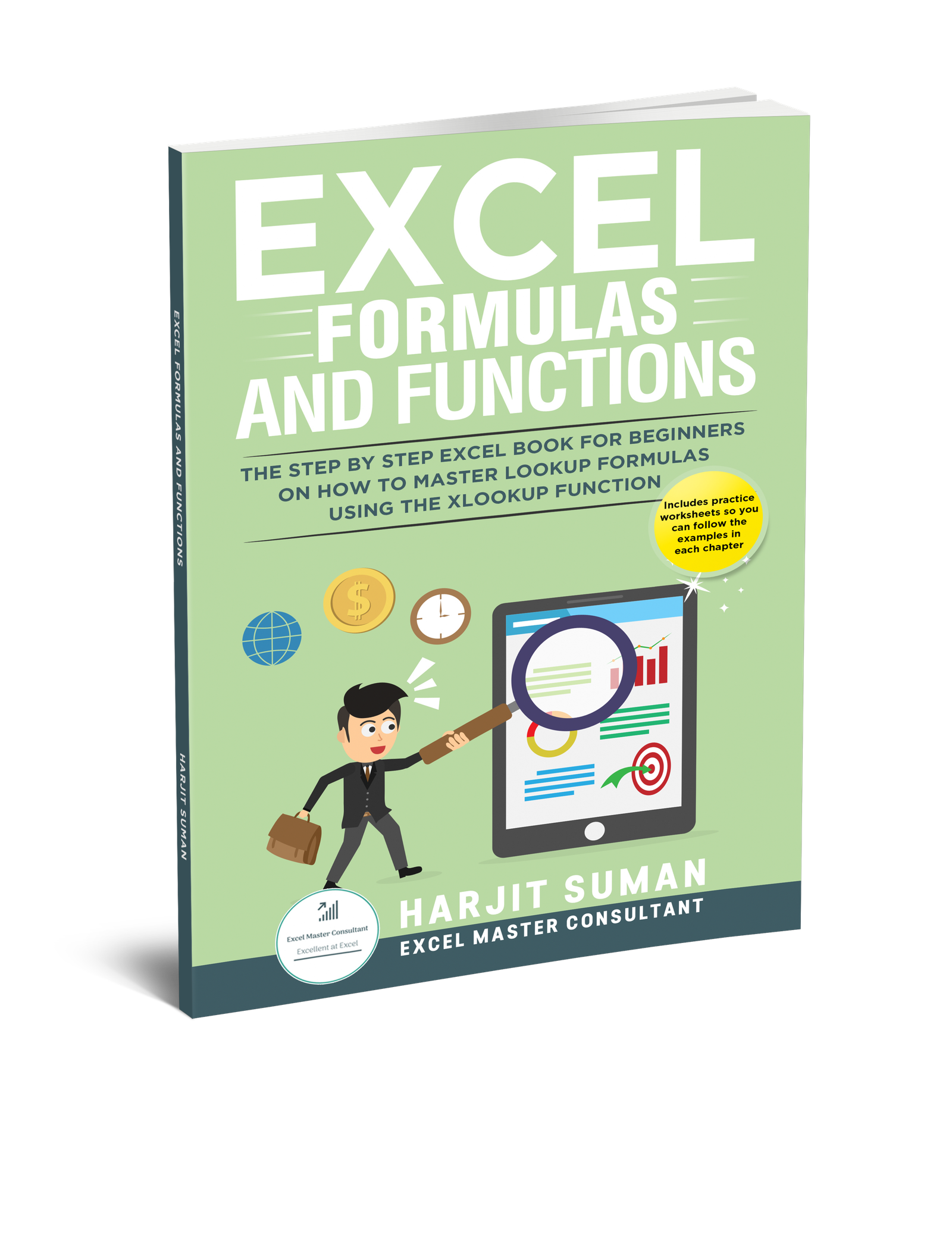# Limitations of VLOOKUP: 5 Big Ones to Know

The VLOOKUP function is one of the most popular functions in Excel. The problem is there are various limitations of VLOOKUP which people are not aware of. This Excel tutorial will highlight what the five big limitations of VLOOKUP are and how you can overcome them.

For those of you who don’t know what VLOOKUP is, you can watch my video tutorial below to learn more about what it is and why it is so useful. You can also read my post on how to use VLOOKUP which will give you examples of how to do an exact and approximate match. After you have read the post, you will be an expert in this very useful function.

## What are the Limitations of the VLOOKUP Function?

So what are the limitations of the VLOOKUP function? I have listed five limitations below which you need to be aware of when you are using VLOOKUP.

### 1. VLOOKUP Only Looks to the Right

One of the biggest limitations is that the VLOOKUP function can only look at the columns to the right of the lookup value.

In this example, there is an employee database which contains information about employees working for a small business. I would like to extract various information by using the employee IDs in column C, however the only information that can be extracted is the department where the employee works in. This is because the department column is to the right of the Employee ID column. You cannot extract the first and last name as this is to the left of the Employee ID column.

In order to extract all the information, the Employee ID column must be the first column in the table, i.e. the left most column in the table. You would have to manually insert the lookup column to the beginning of the table. This is not so bad if you have to do this once but what if there are hundreds of lookup tables in your worksheet which you have to change manually? This will be a very time consuming task to do.

### 2. VLOOKUP Only Finds the First Match

If the lookup value column contains duplicate values then VLOOKUP will only extract the first value.

In the above screenshot, you can see there is a list of sales achieved by Sales people in quarter 1 and 2. In cell F2, there is a VLOOKUP formula to extract the sales for Lee. The problem is that it only extracts the first sales figure which is in quarter 1. If I wanted to extract Lee’s sales in quarter 2 instead, VLOOKUP will not be able to do this. VLOOKUP doesn’t allow you to extract the nth value.

### 3. The Default Setting for VLOOKUP is an Approximate Match

The fourth argument in the VLOOKUP function is the range_lookup. To get an exact match, you either enter FALSE or a 0 in the range_lookup argument. For an approximate match, you enter TRUE or a 1. In most cases you will want to do an exact match. The problem is the fourth argument is an optional argument. If this is omitted, then Excel will use the approximate match. This will cause incorrect results if you want to do an exact match.If the optional fourth argument is omitted you may get incorrect results if you want an exact match lookup

In this example, there is a VLOOKUP formula in cell E2 to extract the Sales person using the sales figure in cell D2 as the lookup value. The fourth argument is omitted so it is doing an approximate match. In an approximate match, VLOOKUP assumes the table is sorted. If VLOOKUP finds a value greater than the lookup value then it will return a value from the previous row. The correct answer should be Kuldeep but VLOOKUP has returned the answer John which is incorrect.

If you want to do an exact match, it is very important that you always remember to make the fourth argument either FALSE or 0.

### 4. VLOOKUP is not Case Sensitive

Another limitation of VLOOKUP is that it doesn’t distinguish between lower and uppercase values. It sees them as the same.

In the above example, there is a list of students with their grades. Note that there are two Jason’s, one is lower case and the other is upper case. In the VLOOKUP formula in cell E2, I would like to extract the grade for “JASON” and not “Jason”. The VLOOKUP formula however cannot distinguish between upper and lower case and because the lower case Jason is before the upper case Jason, it extracts the grade for lower case Jason instead.

### 5. Inserting a Column will Give Incorrect Results

If you insert a column anywhere in the lookup table then the VLOOKUP formula will return an incorrect result. This is because the column number is hard coded in the formula.

In the above example, I have inserted a blank column between the Sales Person column and the Quarter column.

The VLOOKUP formula in cell G2 needs to extract Lee’s sales, however it returns “Quarter 1”. This is because it is looking at the third column, which is now the Quarter column. To fix this, you would have to manually change the third argument in the VLOOKUP formula from 3 to 4 to extract the sales figure for Lee.

To overcome this problem, you can make the third argument dynamic using the COLUMN function. As a result, you don’t have to manually change the third argument each time you add a new column.Use the COLUMN function in the VLOOKUP function’s third argument to ensure you always get the correct results no matter how many columns have been inserted in the lookup table

In the above example, I have changed col_index_num argument from an actual column number to COLUMN()-3. Now, if I insert or delete columns between the Sales Person and the Quarter column, the VLOOKUP function will still return the sales figure.

## How to Overcome the Limitations of VLOOKUP?

Microsoft have released a function called XLOOKUP which overcomes the above VLOOKUP limitations. It is the successor to Excel lookup functions such as VLOOKUP, HLOOKUP, LOOKUP and the INDEX+MATCH functions. Released in 2020, it is only currently available in Office 365. So why have Microsoft released another lookup function when there is already VLOOKUP and HLOOKUP available? XLOOKUP is far more versatile and eliminates the need to use VLOOKUP and HLOOKUP as it does everything these functions can do and more.

I have written a whole book on the topic of the XLOOKUP function. This book called Excel Formulas and Functions: The Step by Step Excel Book for Beginners on how to Master Lookup Formulas using the XLOOKUP Function is available on Amazon now. In this book you will learn what XLOOKUP is, how to perform vertical and horizontal lookups. You will also learn how to replace errors with more meaningful values, how to use wildcard characters to perform partial matches and much more!Excel Formulas and Functions: The Step by Step Excel Book for Beginners on how to Master Lookup Formulas using the XLOOKUP Function

## Wear Your Passion: Explore the Coolest Excel T-Shirts on the Market!

Ready to show off your love for Excel in style? Our collection of Excel-inspired t-shirts is the perfect way to wear your passion proudly. Whether you’re a spreadsheet enthusiast, a data wizard, or just want to make a statement at the office, these tees have got you covered. Don’t miss the chance to elevate your wardrobe and express your data-driven spirit. Click now to explore our selection and find the perfect Excel t-shirt that matches your style!### 2 thoughts on “Limitations of VLOOKUP: 5 Big Ones to Know”

1.Thank you for the appealing read, its not a subject I usually give much attention too but this caught my attention.

1.Thank you for the comment. If you have any questions on this blog or anything else on this website then please let me know.